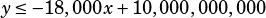KHAN Q:
A memory chip is designed to hold a number of transistors and heat sinks. There must be at least 1 heat sink for every 2000 transistors to prevent overheating. Also, each transistor has an area of 2.0 x 10^-10 mm^2, each heat sink has an area of 3.6 x 10^-6 mm^2, and the total area of transistors and heat sinks must be at most 2 mm^2. What is the approx. max. # of transistors that the chip can hold according to this design?
A. 2.78 x 10^2 B. 5.56 x 10^5 C. 1.0 x 10^9 D. 1.0 x 10^10
Ans=C. S.O.S, PLZ!

Good grief! FWIW, this is one of the Khan Academy questions that really misses the mark for me in terms of similarity to official test questions.

Anyway, let’s translate this into math. We’ll use x for the number of heat sinks and y for the number of transistors.

“There must be at least 1 heat sink for every 2000 transistors…”“each transistor has an area of 2.0 x 10^-10 mm^2, each heat sink has an area of 3.6 x 10^-6 mm^2, and the total area of transistors and heat sinks must be at most 2 mm^2”Use your calculator to evaluate those fractions…Now, some logic. If we’re trying to maximize the number of transistors, then we’re definitely going to use all the available space we have, and the minimal amount of heat sinks, right? So despite the wording of the question, we can avoid persisting with inequalities and just use equalities! (Another way to think about this: if we haveand we’re trying to maximize, we can change it to!)So let’s substitute.Now plug that back into the first equation to get: